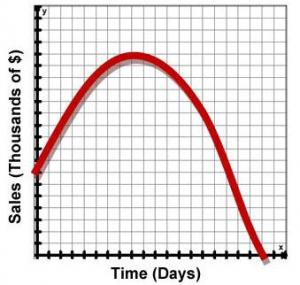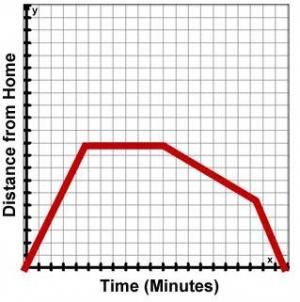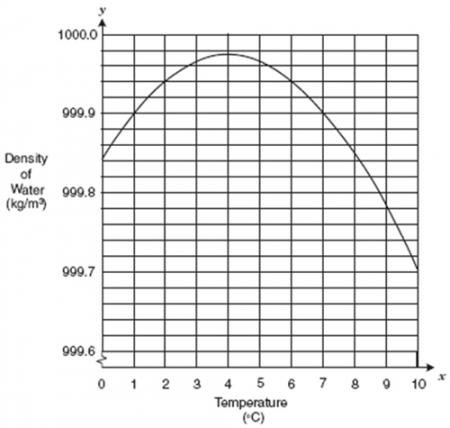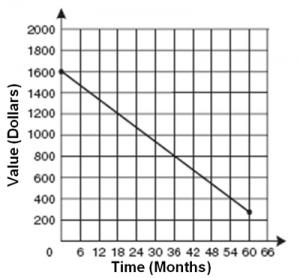# Let's Get Started

Given a graph, you will analyze, interpret, and communicate the mathematical relationship represented and characteristics about this relationship.

TEKS Standards and Student Expectations

A(1) Mathematical process standards. The student uses mathematical processes to acquire and demonstrate mathematical understanding. The student is expected to:

A(1)(E) create and use representations to organize, record, and communicate mathematical ideas

A(1)(F) analyze mathematical relationships to connect and communicate mathematical ideas

Resource Objective(s)

Interpret graphs and create situations based on a given graph.

Essential Questions

What are some common verbs used to represent an increase?

What are some common verbs used to represent a decrease?

What will a graph look like if a variable is increasing

What will a graph look like if a variable is decreasing?

Vocabulary

• Plummet
• Climb
• Half
• Peak
• Fall
• Twice
• Rocket
• Increase
• Appreciate
• Soar
• Depreciate
• Decrease
• Fluctuate
• Descend
• Recede
• Drop
• Ascend
• Rise
• Decline

# Animation of a Biker

We're going to use an interactive tool to both interpret situations in terms of graphs and create situations to fit a graph.

Using an applet called "Animation of a Biker," you can create graphs to match situations. The concepts that can be investigated with the "Animation of a Biker" applet are distance, velocity, and acceleration.

Directions:

1. Click and open the applet in a new window. Note: It will open in a new popup window so that you can toggle between the applet and the directions.
2. Make sure the “Trace Multiple Runs” box is checked beneath the graph.
3. Click on the “Start Animation” button in the lower left hand corner.
4. Change the path by clicking and dragging the points B, C, D, and E, (points A and F are the endpoints).
5. The graph on the right will display the relationship between the speed and position based on the incline the biker is traveling.
6. The “Reset Animation” button moves the biker back to the beginning. The “Reset Ramp” moves the ramp back to the original position.
7. Change the ramps several times while noticing the relationship between the speed and the ramp.

Once you know how to use the applet and have experimented with it, record the following responses in your notes.

Questions:

When the line is horizontal, what is happening?
When the line is decreasing, what is happening?
When the line is increasing, what is happening?
How is this activity linked to creating graphs to fit situations?

# Commonly Used Verbs in Graphs

These verbs can be used to describe common changes represented in linear graphs. Write them down in your notes.

 plummet climb half peak fall twice rocket increase appreciate level out/level off soar depreciate decrease fluctuate descend recede drop ascend rise decline even out

Use an online dictionary to look up the meaning of the verbs, and answer the following questions in your notes. Click below to check your answers.

# Creating Situations for a Given Graph

Using the commonly used words to describe graphs, create a story to match the graph.Using the commonly used words to describe graphs, create a story to match the graph.Using the commonly used words to describe graphs, create a story to match the graph.# Creating Graphs for a Given Situation

Select the graph that represents a possible answer to the given statement for each of the numbered situations below. Click on each graph for feedback on that answer.

Strategies

• Consider the starting point of the scenario as an important part to eliminate graphs.
• Look for key verbs in each sentence.
• When you are looking at the numbers in the graph, use the label's description to help you make sense of what is happening from one point to the next.
• Constant speed means linear.

1. During a recent thunderstorm, the water level in the river increased at a steady rate and remained at a higher level for a period of time. The water then receded at a constant rate nearly to the original level. (Click on each image for feedback the answer.)

2. The pilot decreased the plane's altitude to adjust for turbulence. Once the turbulence was over, the pilot returned the aircraft to its previous cruising altitude. (Click on each image for feedback on that answer.)

3. Diane threw her paper airplane. The airplane lowered at a constant speed, leveled off, and then slowly rose at a constant speed until it was caught by Diane's friend, Ellen. (Click on each image for feedback on that answer.)

# Looking at the Numbers

Strategies

• Mark vertical and horizontal lines representing the numbers given in the problem.
• Calculate a rate (e.g., miles per hour).
• Drawing right angles to represent the horizontal and vertical change will also help.

1. This graph shows the relationship between temperature and the density of water.Which of the following statements best describes this relationship? (Click on each answer for feedback.)

2. Two car rental companies are advertising special rates for a midsize car. Wendell’s Motor Rentals is advertising a rate of $35 a day plus$0.20 per mile traveled, tax included. Marina’s Car Rentals is advertising a rate of $25 a day plus$0.40 per mile traveled, tax included. Which graph correctly compares the cost of renting a midsize car for one day from each company?

3. This graph shows the decrease in the value of a personal computer over a period of 60 months.Which of the following is a reasonable conclusion about the value of this personal computer during the time shown on the graph? (Click on each answer for feedback.)

4. To train for a bicycle road race, Enrique needs to ride 150 miles per week at an average rate of 25 miles per day. To plan his training, Enrique can graph the number of miles, M, left to ride after, D, days. Which graph shows the number of miles Enrique has left to ride after D days? (Click on each graph for feedback.)